# QTL Mapping in Multi-Parent Populations

## The statgenMPP package

The statgenMPP package is developed as an easy-to-use package for QTL mapping in multi-parent populations. The package has many ways of visualizing inputs and results.

This vignette describes in detail how to perform the IBD calculations and how to do QTL mapping using the IBD probabilities in a mixed model framework, using the LMMsolver R package. This will be illustrated using three example data sets. The first data set is a simulated NAM data set with three parents, that is relatively small and runs fast and is mainly used to show the functionality of the package. Then two data sets from literature are used to show the validity of the results from the package, first a maize data set, the dent panel of the EU-NAM maize project (Giraud et al. (2014)). The second data set is a barley data set for awn length described in Liller et al. (2017).

## Theoretical background

### IBD Calculations

The calculations of the IBDs are based on Hidden Markov Models (HMM) and inheritance vectors and are performed using the statgenIBD R package. For details of the theory see Lander and Green (1987) and Huang et al. (2011). It is also possible to import IBD probabilities computed using the RABBIT software . Using the statgenIBD package, IBD probabilities can be calculated for many different types of populations. See the statgenIBD vignette for an overview of these populations. Using RABBIT more complex populations such as MAGIC can also be analyzed.

### QTL Mapping

We follow the methods described in Li et al. (2021) for QTL mapping. In the first step a model without markers is fitted:

$y = X\beta + \varepsilon, \quad \varepsilon \sim { }N\left( {0, \oplus_{k = 1}^{F} I_{{n_{k} }} \sigma_{{\varepsilon_{k} }}^{2} } \right),$ where $$y$$ is a vector with phenotypes, $$X$$ the design matrix for the cross structure, $$\beta$$ a vector of fixed cross intercepts, and the residual term $$\varepsilon$$ has a cross-specific variance covariance structure $$\oplus_{k = 1}^{F} I_{{n_{k} \sigma_{\varepsilon_{k}}^{2}}}$$ in which $$\sigma_{\varepsilon_{k}}^{2}$$ is the residual variance of the $$k^{th}$$ cross with family size $$n_{k}$$, and $$F$$ is the number of families. If $$F=1$$, for example for a single biparental cross or a MAGIC population, the variance covariance structure of the residual term is homogeneous.

Then for each of the evaluation points $$q$$ in the map a second model is fitted:

$y = X\beta + M_{q} a_{q} + \varepsilon, a_{q} \sim { }N\left( {0,I_{P} \sigma_{q}^{2} } \right), \varepsilon \sim { }N\left( {0, \oplus_{k = 1}^{F} I_{{n_{k} }} \sigma_{{\varepsilon_{k} }}^{2} } \right),$ where $$M_{q}$$ is the design matrix containing the expected number of parental alleles as a function of IBD probabilities, $$a_{q}$$ is the vector of random parental effects with variance covariance structure $$I_{P} \sigma_{q}^{2}$$, where $$\sigma_{q}^{2}$$ is the genetic variance of the QTL effects at P parents.

Variance components of a putative QTL from the two models are evaluated to perform the likelihood ratio test (LRT). The p-value is calculated using a $$\chi^2$$ mixture approximation for the Likelihood Ratio Test.

For Multi-QTL Mapping (MQM) the first steps are the same. However after the first round of scanning the QTL with the highest $$-log10(p)$$ value is added as a cofactor in the model and a new round of scanning is done. This is repeated until no new QTLs are found with a $$-log10(p)$$ value above a predefined threshold, or until the predefined maximum number of cofactors is added to the model. In a region of specified width around a cofactor no new cofactors can be detected.

It is possible to include a polygenic $$g$$ term in the models to control for background genetic information. The models fitted then become:

$y = X\beta + g + \varepsilon, \quad g \sim { }N\left( {0,K \sigma_{g}^{2} } \right), \varepsilon \sim { }N\left( {0, \oplus_{k = 1}^{F} I_{{n_{k} }} \sigma_{{\varepsilon_{k} }}^{2} } \right),$

$y = X\beta + M_{q} a_{q} + g + \varepsilon, a_{q} \sim { }N\left( {0,I_{P} \sigma_{q}^{2} } \right), g \sim { }N\left( {0,K \sigma_{g}^{2} } \right), \varepsilon \sim { }N\left( {0, \oplus_{k = 1}^{F} I_{{n_{k} }} \sigma_{{\varepsilon_{k} }}^{2} } \right),$

where the VCOV structure of the polygenic term $$g$$ is $$K \sigma_{g}^{2}$$ with $$\sigma_{g}^{2}$$ the variance of the polygenic effect, and $$K$$ a kinship matrix. To avoid proximal contamination a chromosome specific kinship matrix is used, computed using the markers on the other chromosomes.

The symmetric kinship matrix $$K$$ can have zero eigenvalues, which leads to problems solving the mixed model equations. For that reason we use a spectral decomposition of $$K$$, $$K=U D U^{-1}$$, and only use the eigenvectors $$U_+$$, with corresponding positive eigenvalues in diagonal matrix $$D_+$$. Using the transformation $$g=Zu$$, with $$Z=U_+ D_+^{1/2}$$ we obtain

$y = X\beta + Zu + \varepsilon, \quad u \sim { }N\left( {0,I \sigma_{u}^{2} } \right), \varepsilon \sim { }N\left( {0, \oplus_{k = 1}^{F} I_{{n_{k} }} \sigma_{{\varepsilon_{k} }}^{2} } \right),$

$y = X\beta + M_{q} a_{q} + Zu + \varepsilon, a_{q} \sim { }N\left( {0,I_{P} \sigma_{q}^{2} } \right), g \sim { }N\left( {0,I \sigma_{u}^{2} } \right), \varepsilon \sim { }N\left( {0, \oplus_{k = 1}^{F} I_{{n_{k} }} \sigma_{{\varepsilon_{k} }}^{2} } \right),$

## Example simulated data

As a first example for performing IBD calculations and QTL mapping for a multi-parent population we use a relatively simple simulated data set. The example contains simulated data for two F4DH populations. The population type F4DH is cross between two parents, followed by 3 generations of selfings, followed by a DH generation, see statgenIBD for details.

For the first population the parents where A and B, for the second the parents where A and C. The first population consists of 100 individuals, the second of 80 individuals. This is a simple example of a NAM population, having parent A as central parent. The data is simulated with three QTLs, on chromosome 1, 2, and 3. All necessary data for this population is available in the package.

Before doing QTL detection we compute IBD probabilities on a grid of positions along the genome. This can be done using the calcIBDMPP function in the statgenMPP package. To perform IBD calculations a marker file is required for each of the populations. These files should be a tab-delimited file with first column ID identifying the genotype. The following columns should contain marker information. The first rows should contain the parents used in the cross. As an example, the file for the first cross, AxB, starts like this:

ID M1_1 M1_2 M1_3 M1_4
A 1 2 2 2
B 2 2 2 1
AxB0001 1 2 2 1
AxB0002 2 2 2 2

In this example markers M1_1 and M1_4 are segregating in the AxB cross, M1_2 and M1_3 not.
A map file is also required. This should also be a tab-delimited file with three columns, “marker name”, “chromosome” and “position”. The map file cannot contain a header and has to be identical for all crosses.

Phenotypic data can be added as a data.frame when computing IBD probabilities. Such a data.frame should have a first column “genotype” and all other columns have to be numerical. For the simulated NAM population the data.frame with phenotypic data starts like this:

genotype yield
AxB0001 9.89
AxB0002 6.55
AxB0003 7.90
AxB0004 4.46
AxB0005 5.21
AxB0006 5.27

The phenotypic data only contains a single trait, yield. When performing IBD calculations and specifying phenotypic data, the phenotypic data will be combined with computed IBD probabilities. For this, the genotype specified in the ID column in the marker file(s) will be matched with the genotype in the genotype column in the data.frame with phenotypic information. Phenotypic data for all crosses can either be added from a single data.frame containing phenotypic data for all crosses, or a list of data.frames each containing phenotypic data for a single cross.

## Specify files containing markers.
# One file for each of the two crosses.
markerFiles <- c(system.file("extdata/multipop", "AxB.txt",
package = "statgenMPP"),
system.file("extdata/multipop", "AxC.txt",
package = "statgenMPP"))

## Specify file containing map.
# Both crosses use the same map file.
mapFile <- system.file("extdata/multipop", "mapfile.txt",
package = "statgenMPP")

## Read phenotypic data
phenoDat <- read.delim(system.file("extdata/multipop", "AxBxCpheno.txt",
package = "statgenMPP"))
# Check contents.
#>   genotype yield
#> 1  AxB0001  9.89
#> 2  AxB0002  6.55
#> 3  AxB0003  7.90
#> 4  AxB0004  4.46
#> 5  AxB0005  5.21
#> 6  AxB0006  5.27

## Perform IBD calculations.
ABCMPP <- calcIBDMPP(crossNames = c("AxB", "AxC"),
markerFiles = markerFiles,
pheno = phenoDat,
popType = "F4DH",
mapFile = mapFile,
evalDist = 5)

With calcIBDMPP IBD probabilities are computed on a grid for each of the crosses separately and then combined into a single output object. The value of evalDist can be used to specify the maximum distance (in cM) between two evaluation points on the grid. The exact distance depends on the length of the chromosomes. The output is stored in an object of class gDataMPP (genomic Data for Multi Parent Populations) in which information about map, markers, and phenotypic data is combined. With the summary function we can get some insight in this information.

## Print summary
summary(ABCMPP)
#> map
#>  Number of markers: 95
#>  Number of chromosomes: 5
#>
#> markers
#>  Number of markers: 95
#>  Number of genotypes: 180
#>  Parents: A, B, C
#> pheno
#>  Number of traits: 1
#>  Traitnames: yield
#>  Number of genotypes: 180
#>
#> crosses
#>  AxB:100
#>  AxC: 80

To get a further idea about the population and the computed IBD probabilities we can visualize the results. First we have a look at structure of the pedigree of the population using plotType = "pedigree" to get a general idea of what the design looks like.

## Plot structure of the pedigree.
plot(ABCMPP, plotType = "pedigree")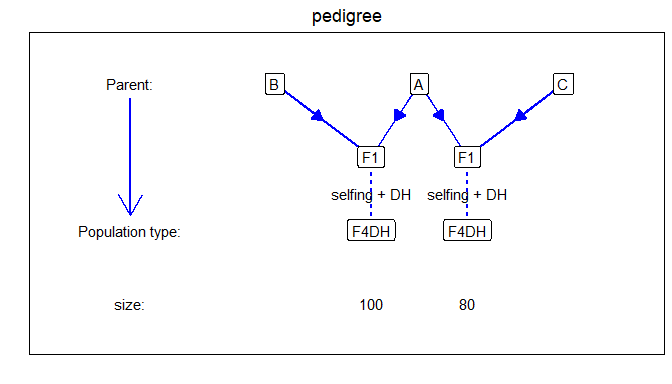Next we look at the genetic map using plotType = "genMap". This will display the genetic map of the population showing the length of each of the chromosomes and indicating the positions where the IBD probabilities were calculated. Optionally it is possible to highlight one or more markers using highlight argument.

## Plot genetic map.
# Highlight marker on chromosome 3 at position 40.
plot(ABCMPP, plotType = "genMap", highlight = "EXT_3_40")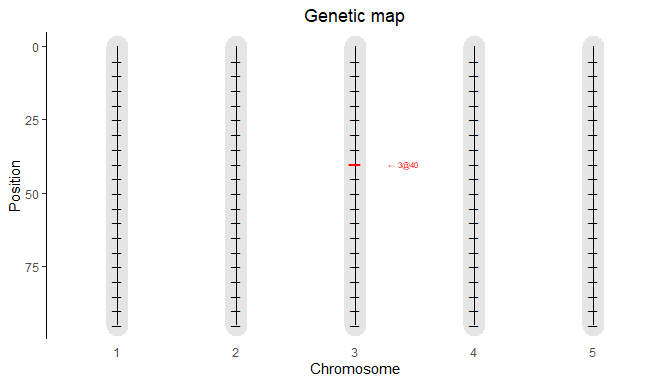Finally we visualize the computed IBD probabilities across the genome for a selected genotype using plotType = "singleGeno". This plot will show the IBD probabilities for all parents for all positions on the genome for the selected genotype.

## Plot IBD probabilities for genotype AxB0001.
plot(ABCMPP, plotType = "singleGeno", genotype = "AxB0001")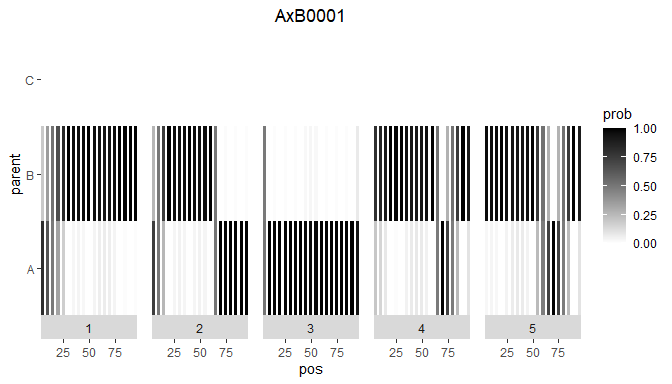Using the computed IBD probabilities we can now do the actual QTL Mapping using the selQTLMPP function. First we perform a SQM by setting maxCofactors = 0. Our trait of interest, yield, is specified in the trait argument of the function.

## Perform Single-QTL Mapping.
ABCSQM <- selQTLMPP(MPPobj = ABCMPP,
trait = "yield",
maxCofactors = 0)

The results of the SQM can be plotted using the plot function with plotType = "QTLProfile".

## Plot QTL Profile for ABC SQM.
plot(ABCSQM, plotType = "QTLProfile")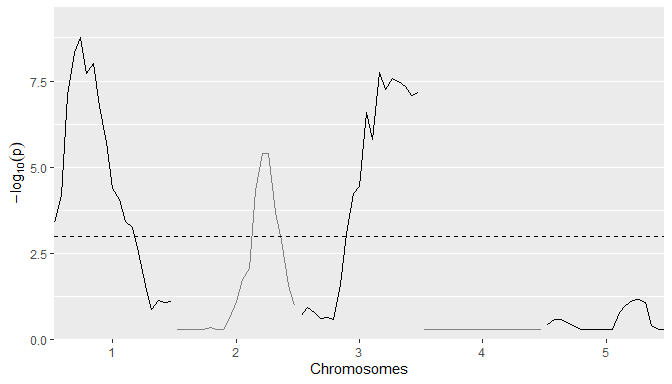Already from the SQM the position of the three simulated QTLs is quite clear. We can get an even better result using MQM. For that, again we use the selQTLMPP function. We don’t specify the maxCofactors to let the algorithm determine the number of cofactors based on the threshold and QTLwindow. As long as new markers are found with a $$-log10(p)$$ value above threshold and the maximum number of cofactors is not reached, a new round of scanning is done. In the new round of scanning the marker with the highest $$-log10(p)$$ value from the previous round is added to the cofactors. Based on the profile plot for the SQM the threshold is set to 3. The QTLwindow is not specified and therefore left at its default value of 10cM.

## Perform Multi-QTL Mapping.
ABCMQM <- selQTLMPP(MPPobj = ABCMPP,
trait = "yield",
threshold = 3)

It is possible to include a polygenic term in the model to control for background genetic information. To do this a list of chromosome specific kinship matrices can either be computed by the selQTLMPP function or specified by the user. In the former case it is enough to specify computeKin = TRUE. When doing so the kinship matrix is computed by averaging $$Z Z^t$$ over all markers, where $$Z$$ is the genotype $$\times$$ parents matrix with IBD probabilities for the marker. A list of precomputed chromosome specific kinship matrices can be specified in K. Note that adding a kinship matrix to the model increases the computation time a lot, especially for large populations. It is advisable to use parallel computing when doing so (see Parallel computing).

## Perform Multi-QTL Mapping.
# Compute kinship matrices.
ABCMQM_kin <- selQTLMPP(MPPobj = ABCMPP,
trait = "yield",
threshold = 3,
computeKin = TRUE)

Next we can visualize the results. First we plot the positions of the QTLs found on the genetic map. This will produce a plot similar the the genetic map plot we have seen before, but now the QTLs will be highlighted. This plot can be made by specifying plotType = "QTLRegion"

## Plot QTL Profile for ABC MQM.
plot(ABCMQM, plotType = "QTLRegion")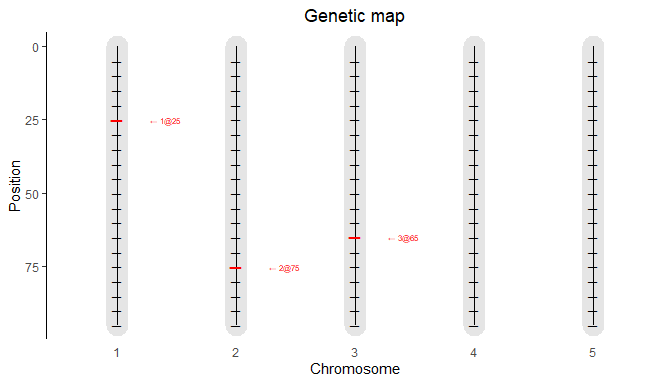As for the SQM we can also plot the QTL profile. The QTLs found will be highlighted in the profile in red.

## Plot QTL Profile for ABC MQM.
plot(ABCMQM, plotType = "QTLProfile")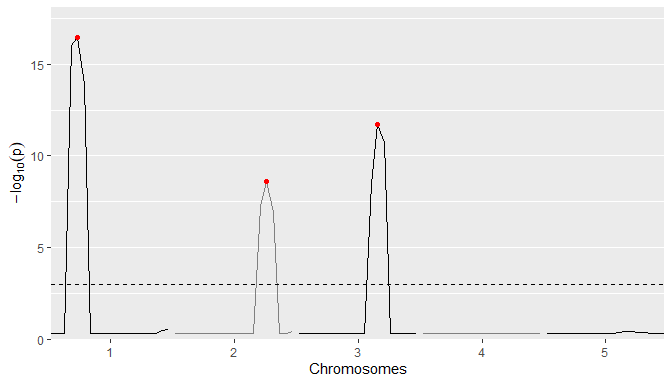It is also possible to plot the size of the parental effects for each of the QTLs found. Positive effects of a parent on the trait will be indicated by shades of red, negative effects by shades of blue. The stronger the color, the larger the effect for the specific parent is. This plot can be made using plotType = "parEffs".

## Plot QTL Profile for ABC MQM.
plot(ABCMQM, plotType = "parEffs")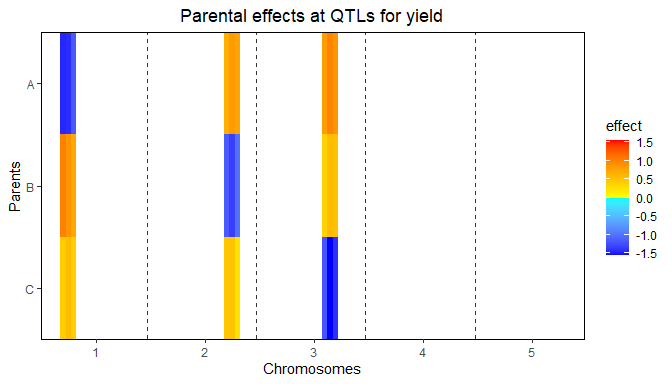Finally a combined plot of the QTL profile and the parental can be made. In this plot the two previous plots are plotted above each other with the chromosomes and positions aligned to allow for easily getting an overview of which effect belongs to which QTL in the QTL profile. This plot can be made using plotType = "QTLProfileExt".

## Plot QTL Profile for ABC MQM.
plot(ABCMQM, plotType = "QTLProfileExt")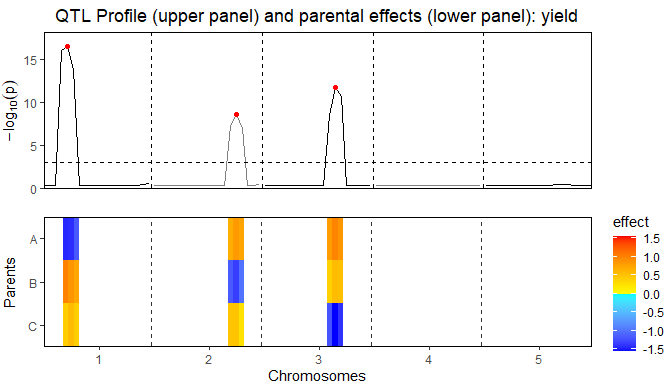A summary of the QTL-analyis gives a short overview containing the total number of markers and the number of QTLs found. Also for all QTL their position on the chromosome is shown as well as the nearest marker on the original map, the explained variance and the effects of all parents.

## Print summary
summary(ABCMQM)
#> Trait analysed: yield
#>
#> Data are available for 95 markers.
#> Threshold: 3
#>
#> Number of QTLs: 3
#>
#>   evalPos chr pos mrkNear minlog10p varExpl  eff_A  eff_B  eff_C
#>  EXT_1_25   1  25    M1_3     16.45   0.242 -1.417  0.859  0.558
#>  EXT_2_75   2  75    M2_8      8.59   0.217  0.833 -1.327  0.495
#>  EXT_3_65   3  65    M3_7     11.69   0.287  0.984  0.560 -1.544

From the output of selQTLMPP the p-Values and effects for all markers can be extracted. They are stored in a data.table within the output object. The example below shows how to extract them. The output will contain columns evalPos, chr and pos with name, chromosome number and evaluation position and columns pValue and eff_par1, eff_par2, eff_par.. with the effects of all parents for that marker.

## Extract results of QTL mapping.
ABCMQMres <- ABCMQM$GWAResult$yield
#> NULL

It is also possible to only extract the markers that are either QTLs or within the window of one of the selected QTLs, as specified when calling the selQTLMPP function. As for the full results, this information in stored in the output as a data.table that can be extracted as shown below. The columns in this data.table are identical to those in the full results except for an additional column at the end, snpStatus, that shows whether a marker is a QTL or within the window of a QTL.

## Extract QTLs and markers within QTL windows.
ABCMQMQTL <- ABCMQM$signSnp$yield
#> NULL

## Example maize

As a second example we use data from a maize NAM population described in Giraud et al. (2014). The NAM population consists of 10 bi-parental doubled haploid (DH) crosses with central parent F353. The total population consists of 841 individuals. Several traits were measured in four locations across Europe. We calculated the best linear unbiased estimations (BLUEs) of those traits using the R package statgenSTA. As an example, we perform QTL mapping for only the mean value of the number of days to silking (“mean_DtSILK”) across all locations. The data for this population is available from the package in zipped format.

As for the simulated data, before doing QTL detection we first compute IBD probabilities on a grid of positions along the genome.

## Define names of crosses.
crosses <- paste0("F353x", c("B73", "D06", "D09", "EC169", "F252", "F618",
"Mo17", "UH250", "UH304", "W117"))
#>  "F353xB73"   "F353xD06"   "F353xD09"   "F353xEC169" "F353xF252"  "F353xF618"

## Specify files containing crosses.
## Extract them in a temporary directory.
tempDir <- tempdir()
crossFiles <- unzip(system.file("extdata/maize/maize.zip", package = "statgenMPP"),
files = paste0(crosses, ".txt"), exdir = tempDir)

## Specify file containing map.
mapFile <- unzip(system.file("extdata/maize/maize.zip", package = "statgenMPP"),
files = "map.txt", exdir = tempDir)

## Read phenotypic data.
phenoFile <- unzip(system.file("extdata/maize/maize.zip", package = "statgenMPP"),
files = "EUmaizePheno.txt", exdir = tempDir)
#>    genotype INR_DMY KWS_DMY Syngenta_DMY TUM_DMY
#> 1 CFD02-003     182      NA          173      NA
#> 2 CFD02-006     172     201          159     243
#> 3 CFD02-010     208     237          159     228
#> 4 CFD02-024     185     219          161     214
#> 5 CFD02-027     206     226          185     221
#> 6 CFD02-036     180     223          163     216

## Perform IBD calculations.
maizeMPP <- calcIBDMPP(crossNames = crosses,
markerFiles = crossFiles,
pheno = phenoDat,
popType = "DH",
mapFile = mapFile,
evalDist = 5)

We then have a look at the summary and some of the plots to get an overview of the pedigree and the computed probabilities.

## Print summary
summary(maizeMPP)
#> map
#>  Number of markers: 262
#>  Number of chromosomes: 10
#>
#> markers
#>  Number of markers: 262
#>  Number of genotypes: 841
#>  Parents: F353, B73, D06, D09, EC169, F252, F618, Mo17, UH250, UH304, W117
#> pheno
#>  Number of traits: 30
#>  Traitnames: INR_DMY, KWS_DMY, Syngenta_DMY, TUM_DMY, ..., mean_NBPL
#>  Number of genotypes: 841
#>
#> crosses
#>  F353xB73  : 64
#>  F353xD06  : 99
#>  F353xD09  :100
#>  F353xEC169: 66
#>  F353xF252 : 96
#>  F353xF618 :104
#>  F353xMo17 : 53
#>  F353xUH250: 94
#>  F353xUH304: 81
#>  F353xW117 : 84
## Plot structure of the pedigree.
plot(maizeMPP, plotType = "pedigree")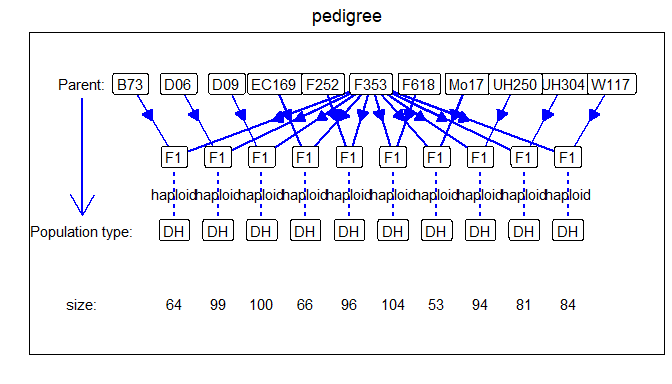Now we can use the computed IBD probabilities to perform SQM.

## Perform Single-QTL Mapping.
maizeSQM <- selQTLMPP(MPPobj = maizeMPP,
trait = "mean_DtSILK",
maxCofactors = 0)

We plot the QTL profile for the SQM to get an idea of reasonable values to use for the threshold and QTLwindow in the MQM that we do next.

## Plot QTL Profile for maize SQM.
plot(maizeSQM, plotType = "QTLProfile")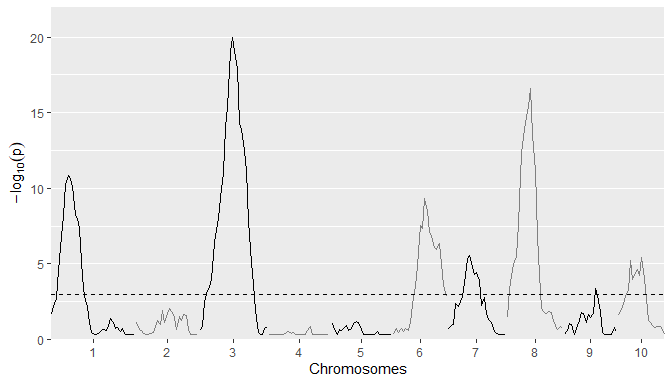Based on the plot for the SQM the threshold is set to 5 to restrict a bit the number of QTLs that will be detected in the MQM. The QTLwindow is not specified and therefore left at its default value of 10cM.

## Perform Multi-QTL Mapping.
maizeMQM <- selQTLMPP(MPPobj = maizeMPP,
trait = "mean_DtSILK",
threshold = 5)

We only look at the combined plot of the QTL Profile and the parental effects. This should give us the most direct insight in the QTLs found and the effects the different parents in the crosses have.

## Plot QTL Profile for maize MQM.
plot(maizeMQM, plotType = "QTLProfileExt")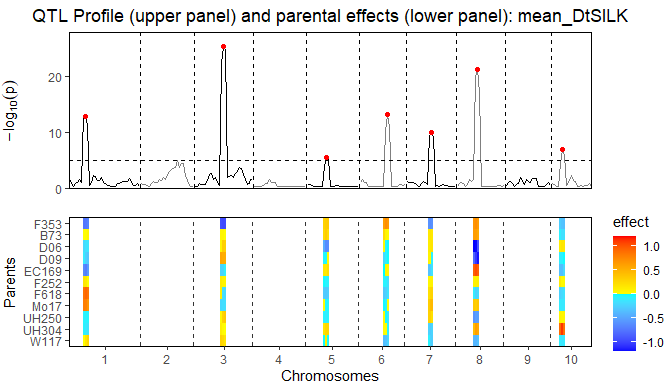## Example barley

Instead of performing IBD calculations directly with the package, it is also possible to import IBD probabilities computed using RABBIT software . The main advantage of using RABBIT for IBD calculations is that it can handle complex pedigree populations and therefore can also be used in cases where the population structure is more complex than those that can be computed using statgenIBD, e.g. in the maize NAM population described before.

As an example we use a barley population described in Liller et al. (2017). This MPP design consists of 5 parents. Four wild parents were crossed with the cultivar Morex and then backcrossed with Morex once. Individuals from the four families from the backcrosses were then crossed with each other as in a full diallel design, which generated six F6 families through five generations of selfing. The trait of interest for this population is awn length (“Awn_length”). As for the maize NAM population, the data for this population is available in zipped format in the package.

RABBIT output can be read using the readRABBITMPP function in statgenMPP. This has as input the standard RABBIT output summary file and the pedigree file that needs to be provided to RABBIT as well. This pedigree file is an optional input and is only used for plotting the pedigree structure of the population. Without it QTL mapping can still be performed. As for calcIBDMPP the phenotypic data has to be provided as a data.frame. This data.frame has been included in the package.

## Specify files containing RABBIT output.
## Extract in a temporary directory.
tempDir <- tempdir()
inFile <- unzip(system.file("extdata/barley/barley_magicReconstruct.zip",
package = "statgenMPP"), exdir = tempDir)

## Specify pedigree file.
pedFile <- system.file("extdata/barley/barley_pedInfo.csv",
package = "statgenMPP")

## Read phenotypic data.
data("barleyPheno")

## read RABBIT output.
barleyMPP <- readRABBITMPP(infile = inFile,
pedFile = pedFile,
pheno = barleyPheno)

As for the maize example we can summarize and plot the imported data to get a first idea of its content.

## Summary.
summary(barleyMPP)
#> map
#>  Number of markers: 355
#>  Number of chromosomes: 7
#>
#> markers
#>  Number of markers: 355
#>  Number of genotypes: 916
#>  Parents: Morex, HID4, HID64, HID369, HID382
#> pheno
#>  Number of traits: 1
#>  Traitnames: Awn_length
#>  Number of genotypes: 916
#>
#> crosses
#>  50:149
#>  51:152
#>  52:157
#>  53:130
#>  54:167
#>  55:161
## Plot structure of the pedigree.
plot(barleyMPP, plotType = "pedigree")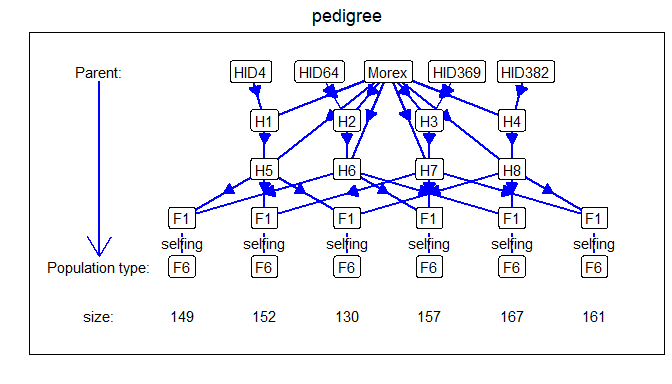Performing SQM and MQM for imported RABBIT output works in the same way as for IBD probabilities computed directly in the package. Since a full scan would take long we precomputed the results and included them in the package.

## Perform Multi-QTL Mapping with threshold 4.
barleyMQM <- selQTLMPP(MPPobj = barleyMPP,
trait = "Awn_length",
threshold = 4)

There is a very large QTL on chromosome 7. To be able to more clearly distinguish the differences between the other QTLs they are plotted separately.

## Plot QTL Profile for barley MQM - chromosome 1-6.
plot(barleyMQM, plotType = "QTLProfileExt", chr = 1:6)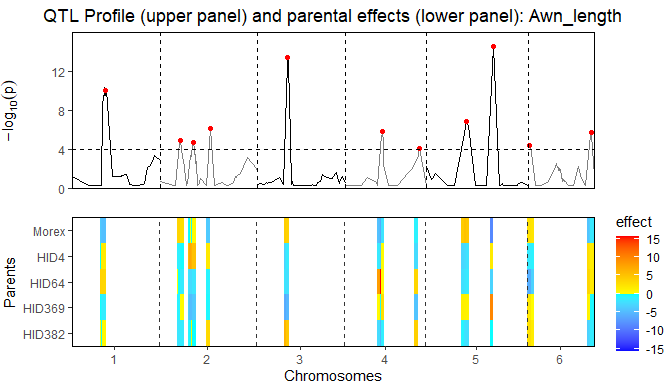## Plot QTL Profile for barley MQM - chromosome 7.
plot(barleyMQM, plotType = "QTLProfileExt", chr = 7)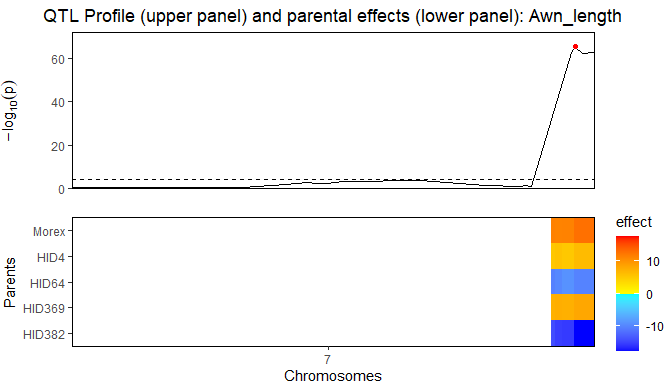The QTLs found are very similar in both position, size and effects as described in Liller et al. (2017). This can also be clearly seen by comparing the summary of the MQM with the table of effects in this paper.

## Summary.
summary(barleyMQM)
#> Trait analysed: Awn_length
#>
#> Data are available for 355 markers.
#> Threshold: 4
#>
#> Number of QTLs: 12
#>
#>  evalPos chr    pos mrkNear minlog10p varExpl eff_Morex eff_HID4 eff_HID64 eff_HID369
#>   2_1053   1  47.06  2_1053     10.05  0.0212   -4.4815     1.72     3.563     -1.425
#>   2_1187   2  27.21  2_1187      4.86  0.0121    3.7109    -1.01    -0.200     -0.815
#>   1_1522   2  46.06  1_1522      4.73  0.0459   -0.0572     7.25    -2.554     -5.226
#>   2_0340   2  68.99  2_0340      6.14  0.0208   -3.8297     1.96    -0.811     -0.437
#>   1_1501   3  42.17  1_1501     13.42  0.0526    4.6614    -2.88    -1.654     -5.951
#>   1_1070   4  51.72  1_1070      5.83  0.0554   -4.7880    -1.89     8.331      0.844
#>   1_0611   4 102.75  1_0611      4.05  0.0299   -0.9099    -2.47     3.531     -4.200
#>   2_0645   5  56.84  2_0645      6.86  0.0268    5.2156    -2.56    -1.824      1.227
#>   1_0094   5  94.26  1_0094     14.48  0.0939   -8.4484     1.70    -3.081      9.928
#>   1_0120   6   1.67  1_0120      4.41  0.0372    2.6349    -1.39    -5.899      1.992
#>   2_0687   6  88.03  2_0687      5.70  0.0344   -4.3277     2.90     3.666      1.144
#>   1_1012   7 121.04  1_1012     65.39  0.2648   11.3779     5.77    -9.658      7.651
#>  eff_HID382
#>       0.629
#>      -1.681
#>       0.583
#>       3.119
#>       5.827
#>      -2.502
#>       4.050
#>      -2.061
#>      -0.102
#>       2.665
#>      -3.387
#>     -15.136

## Parallel computing

To improve performance, it is possible to use parallel computing to perform the QTL mapping. To do this, a parallel back-end has to be specified by the user, e.g. by using registerDoParallel from the doParallel package (see the example below). Besides, in the selQTLMPP function the argument parallel has to be set to TRUE. With these settings the computations are done in parallel per chromosome. Of course, this doesn’t have an effect on the output. The MQM below gives exactly the same results as the non-parallel one described earlier in the vignette.

## Register parallel back-end with 2 cores.
doParallel::registerDoParallel(cores = 2)

## Perform Multi-QTL Mapping.
ABCMQM_Par <- selQTLMPP(MPPobj = ABCMPP,
trait = "yield",
threshold = 3,
parallel = TRUE)

## Summary

The properties of using statgenMPP for QTL mapping in MPPs have been shown with several examples we demonstrated here. This easy-to-use R package, integrating the HMM method for IBD calculation and the mixed-model approach for QTL mapping, provides us with a general framework to estimate multi-allelic QTL effects in terms of parent origins.